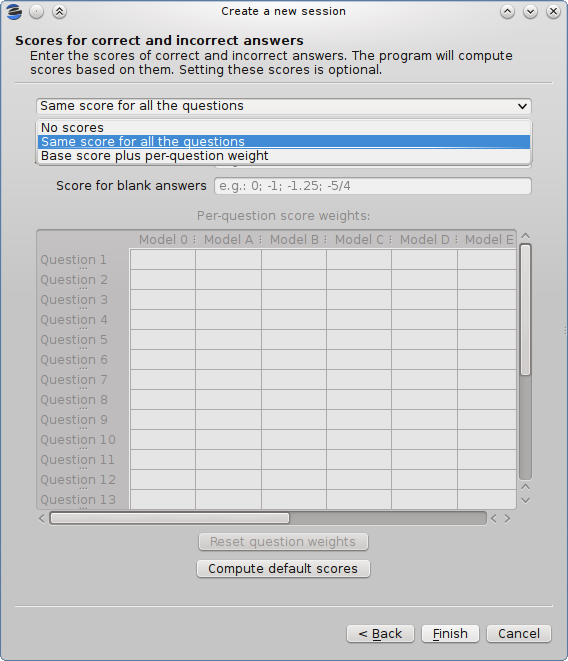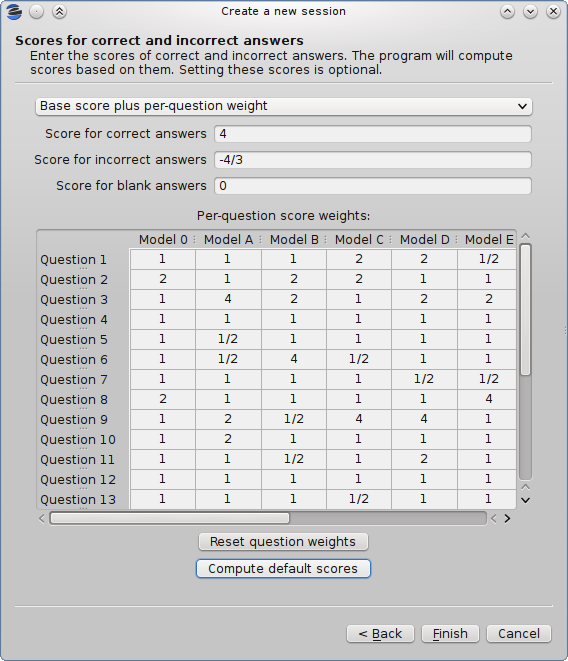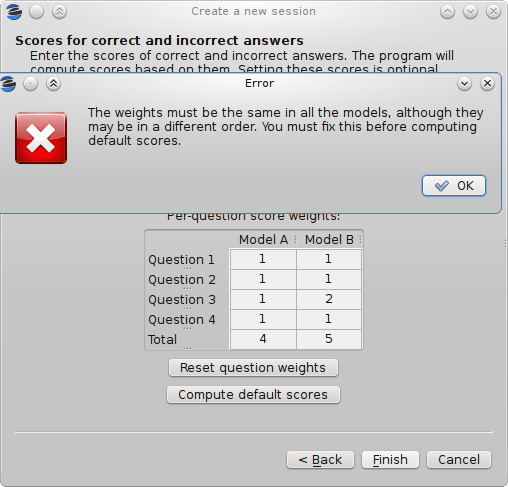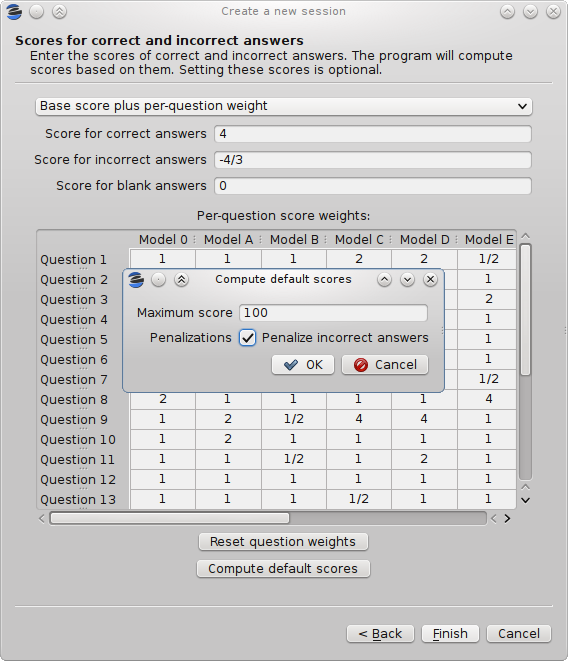# What will eyegrade 0.5 include? (part 3)

Continuing the series of posts that describe the new features of the future eyegrade 0.5 release (see the first and second posts), I'll introduce today a new one: the possibility to assign separate scores to different questions.

Until now, the system assumed that all the questions had the same weight when computing the score for an exam. For example, suppose an exam with 10 questions, in which correct answers add 1 point to the score and incorrect answers subtract 1/3 points (one third of a point). A student having 7 correct and 3 incorrect answers will get 6 points (7 * 1 - 3 * 1/3).

With the new feature, not all the questions need to be awarded the same score. Important questions may get bigger scores than others. In order to do that, you define a base score (e.g. 2 points for correct answers and -2/3 for incorrect ones) and a relative weight for each question. The weight of a question is a factor that multiplies the base score in order to get the actual score of that question. For example, for a question you want to score double than the base score (4 points for correct answers and -4/3 for incorrect ones) you would set a weight of 2. For a question you want to have exactly the base score, you would set a weight of 1. You can even decrease the score of a question with respect to he base score. A weight of 1/2 would mean 1 point for correct answers and -1/3 for incorrect ones in our example.

You'll be able to edit the weights of the questions in one of the steps of the wizard that creates a new session. In that page you select between having no scores, the same score for all the questions and the new weights-based variable score system:If you select the last option, you'll edit the scores in the table at the bottom of the dialog:You can enter in each cell integer numbers (e.g. "2"), fractions (e.g. "1/2") or decimal numbers with fractional digits (e.g. "2.5"). If you have several exam models (alternative orderings of the questions), eyegrade will check that you enter the same weights in all the models, possibly in a different order for each model:In addition, if your session configuration file contains the permutations done to each model (if you create the documents of your exams with eyegrade, it will), eyegrade automatically updates the value in all the models every time you change the weight of a question in one of them. However, if the file does not contain the permutations, you'll need to enter the weights for each model.

I've also updated the dialog that, given a desired maximum score, configures the score for each question. Click on the compute default scores button after you have entered the weights and eyegrade will choose the base score so that the maximum possible grade is the one you choose, taking into account the weights you set:Keep tuned for more updates.